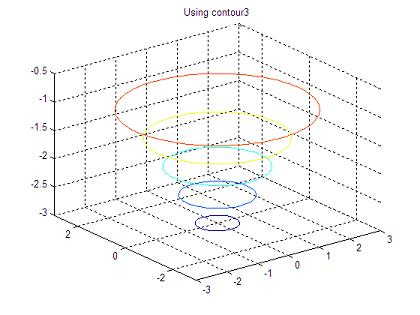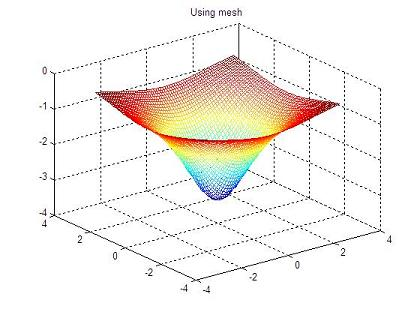# 3D plot – Part 4

Different built-in functions to deal with tridimensional graphs.

 In this example we make a summarization of the use of the following 3D plot instructions: •    meshgrid •    figure •    contour3 •    mesh •    surfc •    surfl It is better if you've already read Parts 1 to 3. We're plotting exactly the same 3D

data (a function depending on two variables) using several instructions, so the visualization is pretty different from each other.

Example:

% cleans the memory workspace
clc; clear; close all;

% defines the range of axes x and y
vx = -3 : 0.1 : 3;
vy = vx;

% generates the powerful grid
[x, y] = meshgrid(vx, vy);

% defines the function to be plotted
z = -10 ./ (3 + x.^2 + y.^2);

% opens figure 1 and plots the function using only contours
figure(1)
contour3(x,y,z)

% opens figure 2 and draws the function using a mesh
figure(2)
mesh(x,y,z)

% opens figure 3 and draws the function using surface with contours
figure(3)
surfc(x,y,z)

% opens figure 4 and draws the function using a enlightened surface
figure(4)
surfl(x,y,z)
colormap hot

The resulting 3D graphics are:From '3D Plot Part 4' to home

From '3D Plot Part 4' to 3D Main